# 深度神经网络 (第七部分)。 神经网络的融合: 堆叠

30 八月 2018, 10:08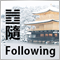0
4 739

### 概述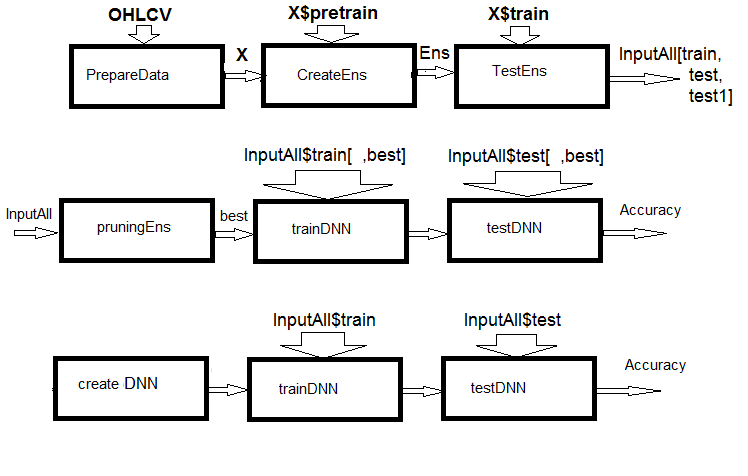• 准备融合的输入数据，训练 ELM 融合并依据训练/测试/测试1 集合获取预测。 这些集合将作为可训练合并器的 InputAll 输入。
• 修剪融合: 按信息重要性选择最佳 ELM 预测。 测试基础比较模块以获取参考度量。 在这些数据上训练和测试 DNN，计算模型的度量并将其与基本模型的度量进行比较。
• 创建一个多模式和多任务神经网络，训练它们并在 InputAll 集合上测试它们。 计算所获模型的度量，并将它们与基本模型的度量进行比较。

### 1. 准备可训练合并器的输入数据

```> library(dplyr)

Attaching package: ‘dplyr’

The following objects are masked from ‘package:stats’:
filter, lag
The following objects are masked from ‘package:base’:
intersect, setdiff, setequal, union
```

```从 theano 导入函数，配置，共享，张量
import numpy as np
import time
```

```#--0--Library-------------
# source(file = "importar.R")
# source(file = "Library.R")
# source(file = "FunPrepareData_VII.R")
# source(file = "FUN_Stacking.R")
#--1-prepare----
evalq({
# combine quotes OHLCV, Med, Typ, W into data frame
# calculate the predictors and the target
dt <- PrepareData(Data, Open, High, Low, Close, Volume)
# split the initial data into pretrain/train/val/test
DT <- SplitData(dt\$feature, 4000, 1000, 500, 250, start = 1)
# define the parameters of outliers
pre.outl <- PreOutlier(DT\$pretrain)
# impute the outliers in all sets
DTcap <- CappingData(DT, impute = T, fill = T, dither = F, pre.outl = pre.outl)
# set the method for normalizing the predictors
meth <- "spatialSign" #"expoTrans" "range" "spatialSign",
# define the normalization parameters
preproc <- PreNorm(DTcap\$pretrain, meth = meth, rang = c(-0.95, 0.95))
# normalize the predictors in all sets
DTcap.n <- NormData(DTcap, preproc = preproc)
}, env)

```

```#--2-Data X-------------
evalq({
foreach(i = 1:length(DTcap)) %do% {
DTcap.n[[i]] ->.;
dp\$select(., Data, ftlm, stlm, rbci, pcci, fars,
v.fatl, v.satl, v.rftl, v.rstl,v.ftlm,
v.stlm, v.rbci, v.pcci, Class)} -> data1
X1 <- vector(mode = "list", 4)
foreach(i = 1:length(X1)) %do% {
data1[[i]] %>% dp\$select(-c(Data, Class)) %>% as.data.frame() -> x
data1[[i]]\$Class %>% as.numeric() %>% subtract(1) -> y
list(x = x, y = y)} -> X1
list(pretrain = X1[] ,
train =  X1[] ,
test =   X1[] ,
test1 =  X1[] ) -> X1
}, env)
#-----------------
evalq({
foreach(i = 1:length(DTcap.n)) %do% {
DTcap.n[[i]] ->.;
dp\$select(., Data, CO, HO, LO, HL, dC, dH, dL)} -> data2
X2 <- vector(mode = "list", 4)
foreach(i = 1:length(X2)) %do% {
data2[[i]] %>% dp\$select(-Data) %>% as.data.frame() -> x
DT[[i]]\$dz -> y
list(x = x, y = y)} -> X2
list(pretrain = X2[] ,
train =  X2[] ,
test =   X2[] ,
test1 =  X2[] ) -> X2
}, env)
```

```#---3--bestF-----------------------------------
#require(clusterSim)
evalq({
orderF(x = X1\$pretrain\$x %>% as.matrix(), type = "metric", s = 1, 4,
distance =  NULL, # "d1" - Manhattan, "d2" - Euclidean,
#"d3" - Chebychev (max), "d4" - squared Euclidean,
#"d5" - GDM1, "d6" - Canberra, "d7" - Bray-Curtis
method = "kmeans" ,#"kmeans" (default) , "single",
#"ward.D", "ward.D2", "complete", "average", "mcquitty",
#"median", "centroid", "pam"
Index = "cRAND") %\$% stopri[ ,1] -> orderX1
}, env)
colnames(env\$X1\$pretrain\$x)[env\$orderX1]
 "v.fatl" "v.rbci" "v.ftlm" "fars"   "v.satl" "stlm"
 "rbci"   "ftlm"   "v.stlm" "v.rftl" "pcci"   "v.rstl"
 "v.pcci
evalq({
orderF(x = X2\$pretrain\$x %>% as.matrix(), type = "metric", s = 1, 4,
distance =  NULL, # "d1" - Manhattan, "d2" - Euclidean,
#"d3" - Chebychev (max), "d4" - squared Euclidean,
#"d5" - GDM1, "d6" - Canberra, "d7" - Bray-Curtis
method = "kmeans" ,#"kmeans" (default) , "single",
#"ward.D", "ward.D2", "complete", "average", "mcquitty",
#"median", "centroid", "pam"
Index = "cRAND") %\$% stopri[ ,1] -> orderX2
}, env)
colnames(env\$X2\$pretrain\$x)[env\$orderX2]
 "dC" "CO" "HO" "LO" "dH" "dL" "HL"
```

• 创建一个 ELM 集合，并在 X1\$pretrain 训练集合上训练它;
• 使用训练的融合预测 X1\$train。 这将是 InputTrain 训练集合。
• 使用训练的融合完成 X1\$test 集合的预测。 这将是 InputTest 测试集合;
• 使用训练的融合完成 X1\$test1 集合的预测。 这将是 InputTest1 测试集合;

```#----Library-------------
import_fun(rminer, holdout, holdout)
#source(file = "FunPrepareData_VII.R")
#----Input-------------
evalq({
#type of activation function.
Fact <- c("sig", #: sigmoid
"sin", #: sine
"hardlim", #: hard-limit
"hardlims", #: symmetric hard-limit
"satlins", #: satlins
"tansig", #: tan-sigmoid
"tribas", #: triangular basis
"poslin", #: positive linear
"purelin") #: linear
n <- 500
#---createENS----------------------
createEns <- function(numFeature = 8L, r = 7L, nh = 5L, fact = 7L, order, X){
# determine the indices of the best predictors
# choose the best predictors for the training set
Xtrain <- X\$pretrain\$x[ , bestF]
k <- 1
rng <- RNGseq(n, 12345)
#---creste Ensemble---
Ens <<- foreach(i = 1:n, .packages = "elmNN") %do% {
rngtools::setRNG(rng[[k]])
idx <- rminer::holdout(Ytrain, ratio = r/10, mode = "random")\$tr
k <- k + 1
elmtrain(x = Xtrain[idx, ], y = Ytrain[idx], nhid = nh, actfun = Fact[fact])
}
return(Ens)
}
#---GetInputData -FUN-----------
GetInputData <- function(Ens, X){
#---predict-InputTrain--
Xtest <- X\$train\$x[ , bestF]
foreach(i = 1:n, .packages = "elmNN", .combine = "cbind") %do% {
predict(Ens[[i]], newdata = Xtest)
} -> predEns #[ ,n]
#---predict--InputTest----
Xtest1 <- X\$test\$x[ , bestF]
foreach(i = 1:n, .packages = "elmNN", .combine = "cbind") %do% {
predict(Ens[[i]], newdata = Xtest1)
} -> InputTest #[ ,n]
#---predict--InputTest1----
Xtest2 <- X\$test1\$x[ , bestF]
foreach(i = 1:n, .packages = "elmNN", .combine = "cbind") %do% {
predict(Ens[[i]], newdata = Xtest2)
} -> InputTest1 #[ ,n]
#---res-------------------------
return(list(InputTrain = predEns,
InputTest = InputTest,
InputTest1 = InputTest1))
}
}, env)
```

```#---4--createEns----------------
evalq({
Ytrain <- X1\$pretrain\$y
Ytest <- X1\$train\$y
Ytest1 <- X1\$test\$y
Ytest2 <- X1\$test1\$y
Ens <- vector(mode = "list", n)
createEns(order = orderX1, X = X1) -> Ens
GetInputData(Ens, X1) -> res
}, env)
```

Result structure:

```> env\$res %>% str()
List of 3
\$ InputTrain: num [1:1001, 1:500] 0.811 0.882 0.924 0.817 0.782 ...
\$ InputTest : num [1:501, 1:500] 0.5 0.383 0.366 0.488 0.359 ...
\$ InputTest1: num [1:251, 1:500] 0.32 0.246 0.471 0.563 0.451 ...
```

### 2. 基础比较模型

```evalq({
getBest <- function(Ens, x, y, nb){
n <- length(Ens)
foreach(i = 1:n, .packages = "elmNN", .combine = "cbind") %do% {
predict(Ens[[i]], newdata = x)} -> y.pr
foreach(i = 1:n, .combine = "c") %do% {
median(y.pr[ ,i])} ->> th
foreach(i = 1:n, .combine = "c") %do% {
ifelse(y.pr[ ,i] > th[i], 1, 0) -> Ypred
Evaluate(actual = y, predicted = Ypred)\$Metrics\$F1 %>%
mean()
} -> Score
Score %>% order(decreasing = TRUE) %>% head(2*nb + 1) -> best
y.pr[ ,best] %>%
apply(1, sum) %>%
divide_by(length(best)) %>%
median() -> med
return(list(Score = Score, bestNN = best, med = med))
}
testAver <- function(Ens, x, y, best, med){
n <- length(Ens)
foreach(i = 1:n, .packages = "elmNN", .combine = "cbind") %:%
when(i %in% best) %do% {
predict(Ens[[i]], newdata = x)} %>%
apply(1, sum) %>% divide_by(length(best)) -> ensPred
ifelse(ensPred > med, 1, 0) -> clAver
Evaluate(actual = y, predicted = clAver)\$Metrics[ ,2:5] %>%
round(3) -> Score
return(list(Score = Score, Ypred = ensPred, clAver = clAver))
}
testVot <- function(Ens, x, y, best){
n <- length(Ens)
foreach(i = 1:n, .packages = "elmNN", .combine = "cbind") %:%
when(i %in% best) %do% {
predict(Ens[[i]], newdata = x)} %>%
apply(2, function(x) ifelse(x > th[i], 1, -1)) %>%
apply(1, function(x) sum(x)) -> vot
ifelse(vot > 0, 1, 0) -> ClVot
Evaluate(actual = y, predicted = ClVot)\$Metrics[ ,2:5] %>%
round(3) -> Score
return(list(Score = Score, Ypred = ClVot))
}
}, env)
```

• 函数 getBest() 返回度量 (Score)，融合的最佳单个分类指数 (bestNN)，融合输出的均值中位数 (med)，并将在测试模型时使用。 所有融合输出的中值向量 th 被插入到环境中。
• 函数 testAver() 返回度量 (Score)，集合的平均连续预测 (Ypred) 和融合的标称预测 (clAver)。
• 函数 testVot() 返回度量 (Score) 和融合的标称预测 (Ypred)。

```#--2---test----
evalq({
Ytrain <- X1\$pretrain\$y
Ytest <- X1\$train\$y
Ytest1 <- X1\$test\$y
Ytest2 <- X1\$test1\$y
Ens <- vector(mode = "list", n)
Ens <- createEns(order = orderX1, X = X1)
#---3------
resBest <- getBest(Ens, x = X1\$train\$x[ , bestF], y = Ytest, nb = 3)
#---4--averaging---
ScoreAver <- testAver(Ens, x = X1\$test\$x[ , bestF], y = Ytest1,
best = resBest\$bestNN, med = resBest\$med)
ScoreAver1 <- testAver(Ens, x = X1\$test1\$x[ , bestF], y = Ytest2,
best = resBest\$bestNN, med = resBest\$med)
#---5--voting----
ScoreVot <- testVot(Ens, x = X1\$test\$x[ , bestF], y = Ytest1,
best = resBest\$bestNN)
ScoreVot1 <- testVot(Ens, x = X1\$test1\$x[ , bestF], y = Ytest2,
best = resBest\$bestNN)
}, env)
> env\$ScoreAver\$Score
Accuracy Precision Recall    F1
0     0.75     0.708  0.778 0.741
1     0.75     0.794  0.727 0.759
> env\$ScoreAver1\$Score
Accuracy Precision Recall    F1
0    0.753     0.750  0.826 0.786
1    0.753     0.758  0.664 0.708
> env\$ScoreVot\$Score
Accuracy Precision Recall    F1
0    0.752     0.702  0.800 0.748
1    0.752     0.808  0.712 0.757
> env\$ScoreVot1\$Score
Accuracy Precision Recall    F1
0    0.741     0.739  0.819 0.777
1    0.741     0.745  0.646 0.692
```

```library(varbvs)
evalq({
vr <- varbvs(X = res\$InputTrain, Z = NULL, y = Ytest,
family = "binomial", optimize.eta = TRUE,
logodds = seq(-6,-2, 0.25), nr = 250,
initialize.params = TRUE,
maxiter = 1e5, verbose = FALSE)
summary(vr, cred.int = 0.95, nv = 7, nr = 1e5) %>% print()
}, env)

family:     binomial   num. hyperparameter settings: 17
samples:    1001       iid variable selection prior: yes
variables:  500        fit prior var. of coefs (sa): yes
covariates: 1          fit approx. factors (eta):    yes
maximum log-likelihood lower bound: -579.4602
Hyperparameters:
estimate Pr>0.95             candidate values
sa          8.09 [7.63,8.54]         NA--NA
logodds    -2.26 [-2.75,-2.00]       (-6.00)--(-2.00)
Selected variables by probability cutoff:
>0.10 >0.25 >0.50 >0.75 >0.90 >0.95
3     3     3     3     3     3
Top 7 variables by inclusion probability:
index variable    prob PVE  coef*  Pr(coef.>0.95)
X18     18      X18 1.00000  NA  4.529 [+3.861,+5.195]
X5       5       X5 1.00000  NA  1.955 [+1.543,+2.370]
X255   255     X255 1.00000  NA  2.097 [+1.537,+2.660]
X109   109     X109 0.00948  NA -1.033 [-2.008,-0.057]
X404   404     X404 0.00467  NA -0.665 [-1.350,+0.024]
X275   275     X275 0.00312  NA -0.726 [-1.735,+0.286]
X343   343     X343 0.00299  NA -0.604 [-1.353,+0.149]
* 请参阅 help(varbvs) 中有关逻辑回归中的解释系数。
```

```env\$vr\$pip %>% order() %>% tail(7) -> bestNN_vr
evalq({
predict(vr, res\$InputTest) -> pr.vr1
Evaluate(actual = Ytest1, predicted = pr.vr1)\$Metrics[ ,2:5] %>%
round(3) -> metr.test
confus(table(Ytest1, pr.vr1)) -> cm1
predict(vr, res\$InputTest1) -> pr.vr2
Evaluate(actual = Ytest2, predicted = pr.vr2)\$Metrics[ ,2:5] %>%
round(3) -> metr.test1
confus(table(Ytest2, pr.vr2)) -> cm2
}, env)
> env\$metr.test
Accuracy Precision Recall    F1
0     0.78     0.750  0.783 0.766
1     0.78     0.808  0.779 0.793
> env\$metr.test1
Accuracy Precision Recall    F1
0    0.729     0.765  0.732 0.748
1    0.729     0.689  0.726 0.707
```

### 3. Keras/TensorFlow 函数库。 一般说明和安装

Keras 是一个高等级神经网络 API。 该软件包的设计重点在于能够快速创建原型，并通过实验测试模型的性能。 以下是 Keras 的主要特点:

• 允许在 CPU 或 GPU 上同等工作。
• 友好的 API，可以轻松创建深度学习模型的原型。
• 内置支持卷积网络 (用于计算机视觉) ，循环网络 (用于处理序列) 及其任意组合。
• 支持任意网络架构: 具有多个输入或多个输出的模型，层共享，模型共享，等等。 这意味着 Keras 基本上适用于构建从存储器网络到神经图灵机的任何深度学习模型。
• 它能够在若干后端的顶层工作，包括 TensorFlow，CNTK 或 Theano

Keras 是为人而不是机器设计的 API。 该软件包降低了认知负担: 它提供了一致且简单的 API，最大限度地减少了用户操作的数量，并提供了有关用户错误的有效反馈。 所有这些令 Keras 易于学习和使用。 但这不是靠降低灵活性造成的: 因为 Keras 与深度学习的低级语言 (特别是 TensorFlow) 集成，它允许您实现在基础语言上可创建的所有东西。

• CNTK 后端 (来 Microsoft)
• Theano 后端

Keras 和 TensorFlow 可配置在 CPU 或 GPU 上运行。 CPU 版本更易于安装和设置，因此，它是开始使用该软件包的最佳选择。 以下是 TensorFlow 网站上 CPU 和 GPU 版本的手册:

• TensorFlow 仅支持 CPU. 如果您的系统没有 NVIDIA® GPU，则必须安装此版本。
• TensorFlow 仅支持 GPU. TensorFlow 程序在 GPU 上运行通常比在 CPU 上运行快得多。 因此，如果您的系统具有满足所有先决条件的 NVIDIA® GPU，并且您需要运行性能优先的应用程序，则最终应安装此版本。

Windows 上唯一受支持的安装方法是 "conda"。 这意味着您应该在安装 Keras 之前为 Windows 安装 Anaconda 3.x (Python 3.5.x/3.6.x)。 我安装了 Anaconda3(Python3.6)

`install.packages("keras")`

Keras R 接口默认使用 TensorFlow。 若要安装 Keras 主体函数库和 TensorFlow 后端，请使用 install_keras() 函数:

```# default installation
library(keras)
install_keras()
```

```# install with GPU version of TensorFlow
# (NOTE: only do this if you have an NVIDIA GPU + CUDA!)
install_keras(tensorflow = "gpu")

# install a specific version of TensorFlow
install_keras(tensorflow = "1.5")
install_keras(tensorflow = "1.5-gpu")
```

tfruns 支持的软件包用于 TensorFlow 的实验。 这是一套用于管理来自 R 的 TensorFlow 训练和实验的工具包。

• 跟踪每次训练运行的超参数，度量，输出数据和源代码。
• 比较运行中的超参数和度量，以便找到性能最佳的模型。
• 自动生成报告以便可视化各单一训练运行或所有运行之间的比较。
• 不需要更改源代码 (为所有 Keras 和 tfestimators 模型自动捕获运行数据)。

DNN 训练过程和结果的最佳可视化品质由 TensorBoard 提供。

```> library(reticulate)
> py_config()
python:         K:\Anaconda3\envs\r-tensorflow\python.exe
libpython:      K:/Anaconda3/envs/r-tensorflow/python36.dll
pythonhome:     K:\ANACON~1\envs\R-TENS~1
version:        3.6.5 | packaged by conda-forge | (default, Apr  6 2018, 16:13:55)
[MSC v.1900 64 bit (AMD64)]
Architecture:   64bit
numpy:          K:\ANACON~1\envs\R-TENS~1\lib\site-packages\numpy
numpy_version:  1.14.2
tensorflow:     K:\ANACON~1\envs\R-TENS~1\lib\site-packages\tensorflow

python versions found:
K:\Anaconda3\envs\r-tensorflow\python.exe
K:\ANACON~1\python.exe
K:\Anaconda3\python.exe
```

```> library(tensorflow)
> tf_config()
TensorFlow v1.5.1 (K:\ANACON~1\envs\R-TENS~1\lib\site-packages\tensorflow)
Python v3.6 (K:\Anaconda3\envs\r-tensorflow\python.exe)

```

### 4. 袋型融合输出合并器 — 神经网络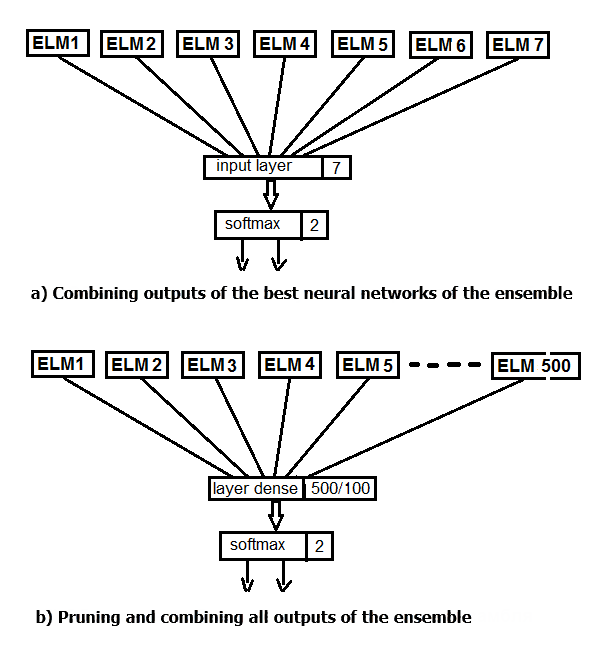Keras 的主要数据结构是一种模型，一种组织层的方式。 最简单的类型是 顺序模型，表示层的线性堆叠。

```#===========Keras===========================================
library(keras)

num_classes <- 2L
batch_size <- 32L
epochs <- 300L
#---------
bestNN <- env\$resBest\$bestNN
x_train <- env\$res\$InputTrain[ ,bestNN]
y_train <- env\$Ytest %>% to_categorical()
x_test <- env\$res\$InputTest[ ,bestNN]
y_test <- env\$Ytest1 %>% to_categorical()
x_test1 <- env\$res\$InputTest1[ ,bestNN]
y_test1 <- env\$Ytest2 %>% to_categorical()
```

```##----model--keras-------------------------
# define model
model <- keras_model_sequential()

model %>%
layer_dense(units = num_classes, input_shape = dim(x_train)) %>%
layer_activation(activation = 'softmax') %>%
compile(
loss = 'binary_crossentropy',
optimizer =  optimizer_rmsprop(),
metrics = 'accuracy'
)
```

• 没有必要将每次迭代的结果输出到终端;
• 没有必要在 Viewer/Rstudio 中显示实时图表;
• 有必要在每个训练期之后对输入数据进行筛洗。

```## Training & Evaluation ---------------------------
# Fit model to data
model %>% fit(
x_train, y_train,
batch_size = batch_size,
epochs = epochs,
verbose = 0,
view_metrics = FALSE,
shuffle = TRUE,
validation_split = 0.2) -> history
# Output metrics
score <- model %>% evaluate(x_test, y_test, verbose = 0)
cat('Test loss:', score[] %>% round(3), '\n')
Test loss: 0.518
cat('Test accuracy:', score[] %>% round(3), '\n')
Test accuracy: 0.754
#---------------
score1 <- model %>% evaluate(x_test1, y_test1, verbose = 0)
cat('Test loss:', score1[] %>% round(3), '\n')
Test loss: 0.55
cat('Test accuracy:', score1[] %>% round(3), '\n')
Test accuracy: 0.737
```

```#--plot------------------------
plot(history)
```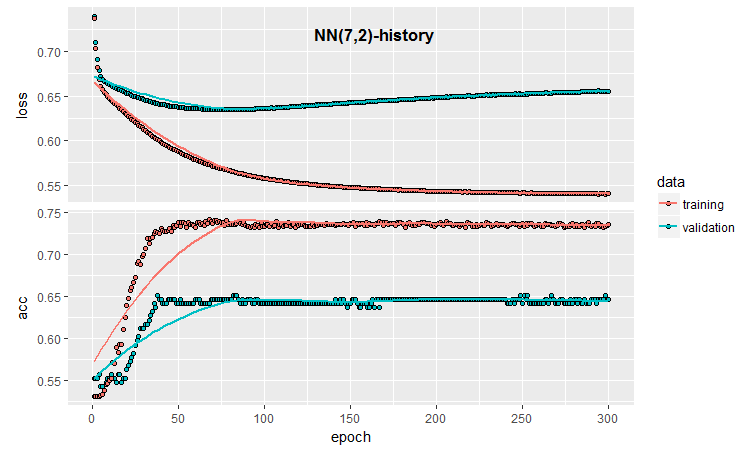```callback_early_stopping(monitor = "val_loss", min_delta = 0, patience = 0,
verbose = 0, mode = c("auto", "min", "max"))
callback_tensorboard(log_dir = NULL, histogram_freq = 0, batch_size = 32,
write_graph = TRUE, write_grads = FALSE, write_images = FALSE,
embeddings_freq = 0, embeddings_layer_names = NULL,
```

```early_stopping <- callback_early_stopping(monitor = "val_acc", min_delta = 1e-5,
patience = 20, verbose = 0,
mode = "auto")
log_dir <- paste0(getwd(),"/run_1")
tensboard <- callback_tensorboard(log_dir = log_dir, histogram_freq = 1,
batch_size = 32, write_graph = TRUE,
write_grads = TRUE, write_images = FALSE)
```

```##=====Variant  earlystopping=================================
#--prepare data--------------------------
library(reticulate)
library(keras)
py_set_seed(12345)

num_classes <- 2L
batch_size <- 32L
learning_rate <- 0.005
epochs <- 100L
#---------
bestNN <- env\$resBest\$bestNN
x_train <- env\$res\$InputTrain[ ,bestNN]
y_train <- env\$Ytest %>% to_categorical()
x_test <- env\$res\$InputTest[ ,bestNN]
y_test <- env\$Ytest1 %>% to_categorical()
x_test1 <- env\$res\$InputTest1[ ,bestNN]
y_test1 <- env\$Ytest2 %>% to_categorical()
##----model--keras-------------------------
# define model
model <- keras_model_sequential()
model %>%
layer_gaussian_noise(stddev = 0.05, input_shape = dim(x_train),
name = "GN") %>%
layer_dense(units = num_classes, name = "dense1") %>%
layer_activation_softmax(name = "soft") %>%
layer_activity_regularization(l2 = 1.0, name = "reg") %>%  #l1 = 0.01,
compile(
loss = 'binary_crossentropy',
optimizer =  optimizer_rmsprop(lr = learning_rate, decay = 0.01),
metrics = 'accuracy'
)
## Training & Evaluation ---------------------------
# Fit model to data
model %>% fit(
x_train, y_train,
batch_size = batch_size,
epochs = epochs,
verbose = 0,
view_metrics = TRUE ,
shuffle = TRUE,
validation_split = 0.2,
callbacks = list(early_stopping, tensboard)) -> history
```

```# Output metrics
> score <- model %>% evaluate(x_test, y_test, verbose = 0)
> cat('Test loss:', score[] %>% round(3), '\n')
Test loss: 0.539
> cat('Test accuracy:', score[] %>% round(3), '\n')
Test accuracy: 0.756
> #---------------
> score1 <- model %>% evaluate(x_test1, y_test1, verbose = 0)
> cat('Test loss:', score1[] %>% round(3), '\n')
Test loss: 0.571
> cat('Test accuracy:', score1[] %>% round(3), '\n')
Test accuracy: 0.713

```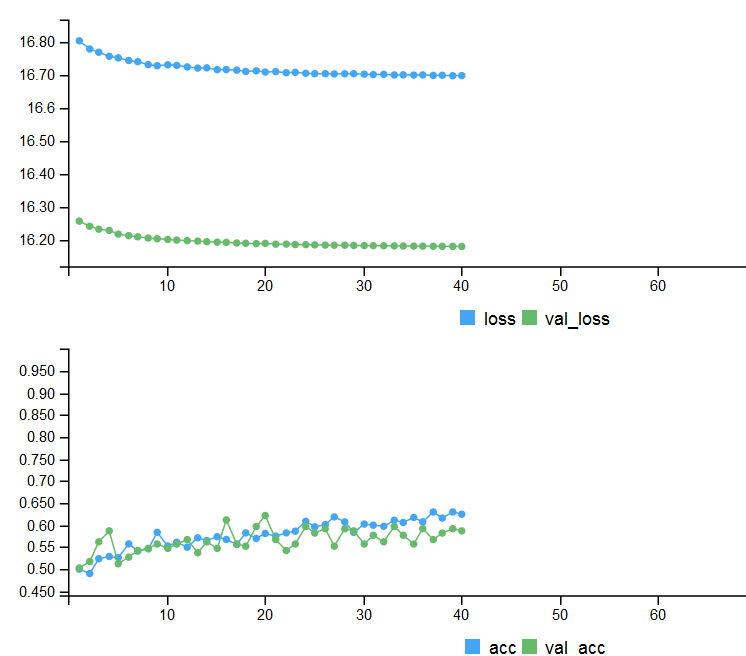```> tensorboard(log_dir = log_dir)
TensorBoard 1.7.0 at http://127.0.0.1:7451 (Press CTRL+C to quit)
Started TensorBoard at http://127.0.0.1:7451
```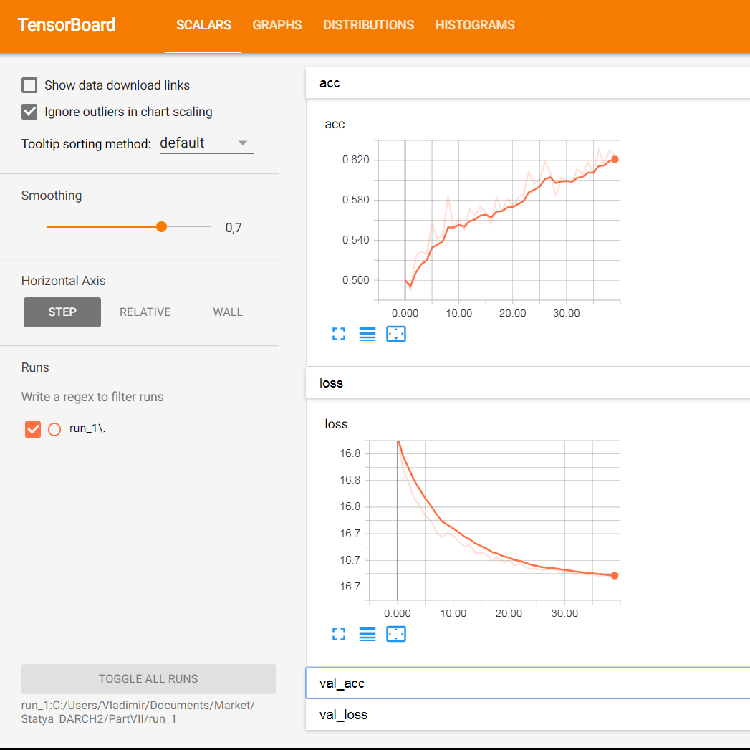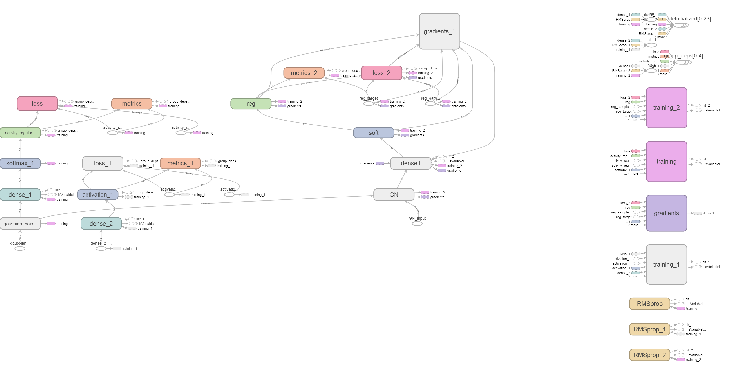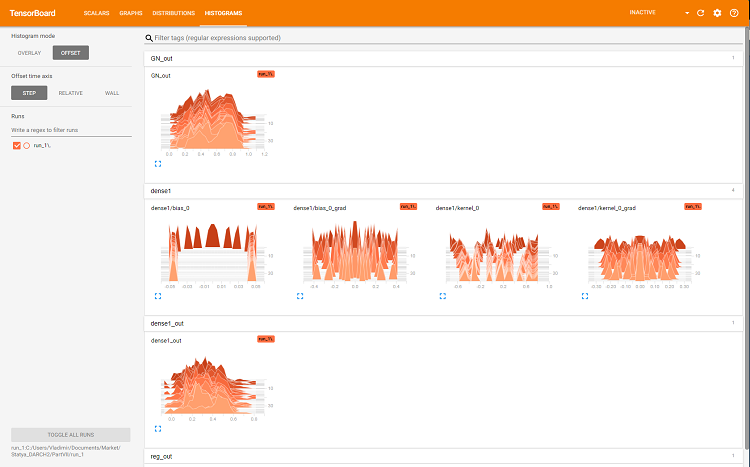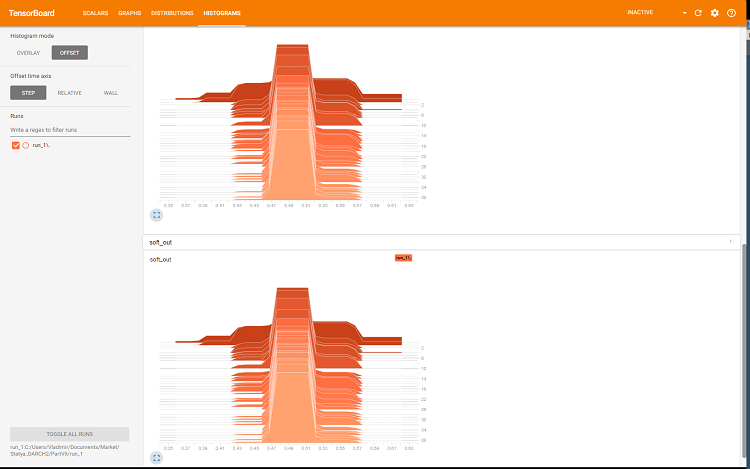```library(reticulate)
library(keras)
py_set_seed(12345)

num_classes <- 2L
batch_size <- 32L
learning_rate <- 0.005
epochs <- 100L
#---------
bestNN <- env\$resBest\$bestNN
x_train <- env\$res\$InputTrain[ ,bestNN]
y_train <- env\$Ytest %>% to_categorical()
x_test <- env\$res\$InputTest[ ,bestNN]
y_test <- env\$Ytest1 %>% to_categorical()
x_test1 <- env\$res\$InputTest1[ ,bestNN]
y_test1 <- env\$Ytest2 %>% to_categorical()
#----------------------------------------
early_stopping <- callback_early_stopping(monitor = "val_acc", min_delta = 1e-5,
patience = 20, verbose = 0,
mode = "auto")
log_dir <- paste0(getwd(),"/run_2")
tensboard <- callback_tensorboard(log_dir = log_dir, histogram_freq = 1,
batch_size = 32, write_graph = TRUE,
write_grads = TRUE, write_images = FALSE)
##----model--keras-------------------------
# define model
model <- keras_model_sequential()
model %>%
layer_gaussian_noise(stddev = 0.05, input_shape = dim(x_train),
name = "GN") %>%
layer_dense(units = num_classes, name = "dense1") %>%
layer_activation_softmax(name = "soft") %>%
layer_activity_regularization(l2 = 1.0, name = "reg") %>%  #l1 = 0.01,
compile(
loss = 'binary_crossentropy',
optimizer =  optimizer_rmsprop(lr = learning_rate, decay = 0.01),
metrics = 'accuracy'
)
## Training & Evaluation ---------------------------
# Fit model to data
model %>% fit(
x_train, y_train,
batch_size = batch_size,
epochs = epochs,
verbose = 0,
view_metrics = TRUE ,
shuffle = TRUE,
validation_data = list(x_test, y_test),
callbacks = list(early_stopping, tensboard)) -> history
```

让我们看看第二个测试集合上的度量:

```#--model--test1-------------------------------------------------
predict(model, x_test1) -> Ypr.test1
Ypr.test1 %>% max.col()-1 -> y_pr_test1
#Ypr.test1 %>% apply(1, function(x) which.max(x)) %>% subtract(1) -> y_pr_test1
evalq(res_mod_test1 <- Eval(Ytest2, y_pr_test1), env)
> env\$res_mod_test1
\$metrics
Accuracy Precision Recall    F1
0    0.713     0.704  0.826 0.760
1    0.713     0.730  0.575 0.644

\$confMatr
Confusion Matrix and Statistics

predicted
actual   0   1
0 114  24
1  48  65

Accuracy : 0.7131
95% CI : (0.6529, 0.7683)
No Information Rate : 0.6454
P-Value [Acc > NIR] : 0.013728

Kappa : 0.4092
Mcnemar's Test P-Value : 0.006717

Sensitivity : 0.7037
Specificity : 0.7303
Pos Pred Value : 0.8261
Neg Pred Value : 0.5752
Prevalence : 0.6454
Detection Rate : 0.4542
Detection Prevalence : 0.5498
Balanced Accuracy : 0.7170

'Positive' Class : 0
```

``` #-----plot------------------
tensorboard(log_dir = c(paste0(getwd(),"/run_1"), paste0(getwd(),"/run_2")))
```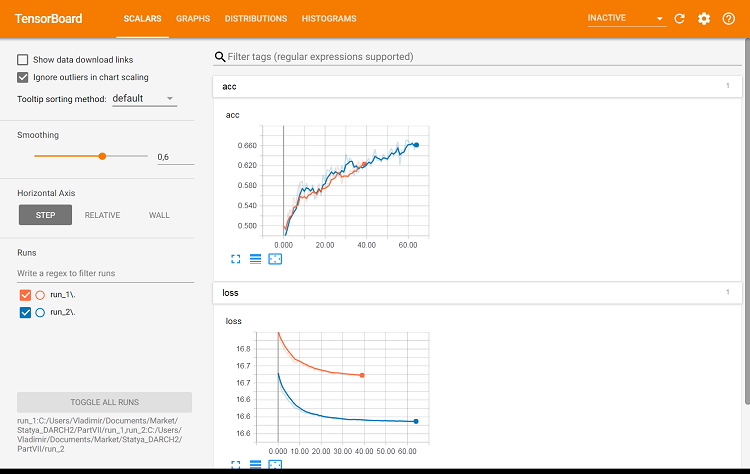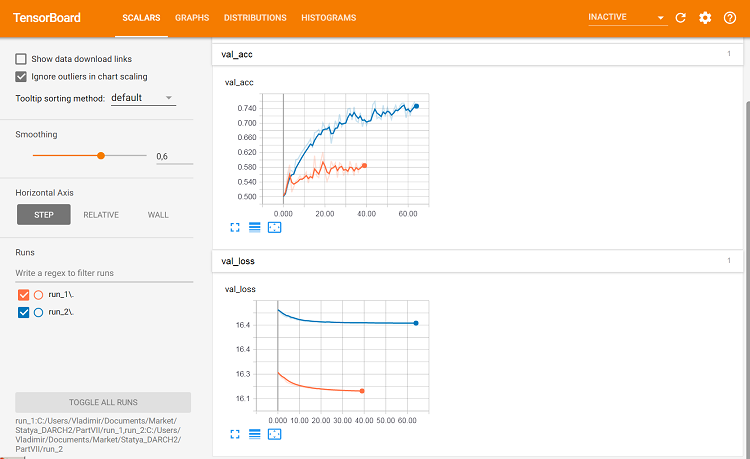```library(reticulate)
library(keras)
py_set_seed(12345)

num_classes <- 2L
batch_size <- 32L
learning_rate <- 0.0001
epochs <- 100L
#---------
x_train <- env\$res\$InputTrain
y_train <- env\$Ytest %>% to_categorical()
x_test <- env\$res\$InputTest
y_test <- env\$Ytest1 %>% to_categorical()
x_test1 <- env\$res\$InputTest1
y_test1 <- env\$Ytest2 %>% to_categorical()
#----------------------------------------
early_stopping <- callback_early_stopping(monitor = "val_acc", min_delta = 1e-5,
patience = 20, verbose = 0,
mode = "auto")

```

```##----modelDNN--keras-------------------------
# define model
modDNN <- keras_model_sequential()
modDNN %>%
layer_gaussian_noise(stddev = 0.001, input_shape = dim(x_train), name = "GN") %>%
layer_batch_normalization() %>%
layer_dense(units = 100, activation = "elu", name = "dense1") %>%
layer_dropout(rate = 0.5, name = "dp1") %>%
layer_batch_normalization() %>%
layer_dense(units = 50, activation = "elu", name = "dense2") %>%
layer_batch_normalization() %>%
layer_dropout(rate = 0.5, name = "dp2") %>%
layer_dense(units = 10, activation = "elu", name = "dense3") %>%
layer_batch_normalization() %>%
layer_dropout(rate = 0.2, name = "dp3") %>%
layer_dense(units = num_classes, activation = "softmax", name = "soft") %>%
compile(
loss = 'binary_crossentropy',
optimizer =  optimizer_rmsprop(lr = learning_rate, decay = 0.0001),
metrics = 'accuracy'
)
```

```## Training & Evaluation ---------------------------
# Fit model to data
modDNN %>% fit(
x_train, y_train,
batch_size = batch_size,
epochs = epochs,
verbose = 0,
view_metrics = TRUE ,
shuffle = TRUE,
validation_split = 0.2,
#validation_data = list(x_test, y_test),
callbacks = list(early_stopping)) -> history
```

```#--model--test-------------------------
predict(modDNN, x_test) -> Ypr.test
Ypr.test %>% apply(1, function(x) which.max(x)) %>% subtract(1) -> y_pr_test
evalq(res_mod_test <- Eval(Ytest1, y_pr_test), env)
```

```> env\$res_mod_test
\$metrics
Accuracy Precision Recall    F1
0    0.752     0.702  0.800 0.748
1    0.752     0.808  0.712 0.757

\$confMatr
Confusion Matrix and Statistics

predicted
actual   0   1
0 184  46
1  78 193

Accuracy : 0.7525
95% CI : (0.7123, 0.7897)
No Information Rate : 0.523
P-Value [Acc > NIR] : < 2.2e-16

Kappa : 0.5068
Mcnemar's Test P-Value : 0.005371

Sensitivity : 0.7023
Specificity : 0.8075
Pos Pred Value : 0.8000
Neg Pred Value : 0.7122
Prevalence : 0.5230
Detection Rate : 0.3673
Detection Prevalence : 0.4591
Balanced Accuracy : 0.7549

'Positive' Class : 0
```

`plot(history)`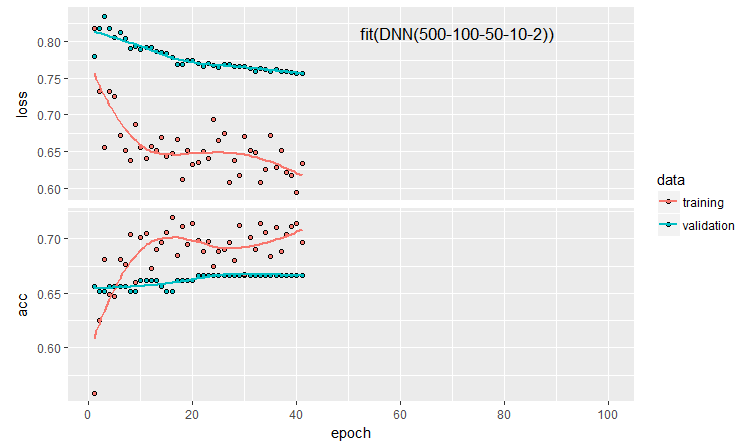### 5. 实验结果分析

• 均值融合 (EnsAver);
• 多数表决融合 (EnsVot);
• 逻辑回归模型变体;
• 神经网络 DNN(7,2);
• 神经网络 DNN500。

```#---bias--test-------------------------------
import_fun(randomUniformForest, biasVarCov, BiasVar)
evalq({
target = Ytest1
biasAver <- BiasVar(predictions = ScoreAver\$clAver,
target = target,
regression = FALSE, idx = 1:length(target))
biasVot <- BiasVar(predictions = ScoreVot\$ClVot,
target = target,
regression = FALSE, idx = 1:length(target))
}, env)
-----------------------------
Noise: 0.2488224
Squared bias: 0.002107561
Variance of estimator: 0.250475
Covariance of estimator and target: 0.1257046

Assuming binary classification with classes {0,1}, where '0' is the majority class.
Misclassification rate = P(Y = 1)P(Y = 0) + {P(Y = 1) - P(Y_hat = 1)}^2 + P(Y_hat = 0)P(Y_hat = 1) - 2*Cov(Y, Y_hat)
Misclassification rate = P(Y = 1) + P(Y_hat = 1) - 2*E(Y*Y_hat) = 0.2499958
---------------------
Noise: 0.2488224
Squared bias: 0.004079665
Variance of estimator: 0.2499721
Covariance of estimator and target: 0.1274411

Assuming binary classification with classes {0,1}, where '0' is the majority class.
Misclassification rate = P(Y = 1)P(Y = 0) + {P(Y = 1) - P(Y_hat = 1)}^2 + P(Y_hat = 0)P(Y_hat = 1) - 2*Cov(Y, Y_hat)
Misclassification rate = P(Y = 1) + P(Y_hat = 1) - 2*E(Y*Y_hat) = 0.2479918
```

```> env\$biasAver
\$predError
 0.2499958

\$squaredBias
 0.002107561

\$predictionsVar
 0.250475

\$predictionsTargetCov
 0.1257046
```

95%CI Acc Precision Recall  F1 PredErr sqBias  predVar predTargCov
EnsAver  0.7102, 0.7880 (0.7500) 0.708
0.794
0.778
0.727
0.741
0.759
0.2499 0.0021  0.2505 0.1257
EnsVot 0.7123, 0.7897 (0.7525) 0.702
0.808
0.800
0.712
0.748
0.757
0.248 0.0041  0.25 0.1274
varb 0.7416, 0.8159 (0.7804) 0.790
0.808
0.783
0.779
0.766
0.793
0.2199 0.000398  0.25 0.13964
DNN(7, 2) 0.7165, 0.7935 (0.7565) 0.765
0.751
0.678
0.823
0.719
0.785
0.2460 0.000195  0.2498 0.1264
DNN500 0.7123, 0.7897 (0.7525) 0.702
0.808
0.800
0.712
0.748
0.757
0.2779 0.01294  0.2452 0.1145

1. 基本模型中的最佳 Accuracy (准确度) 得分由合并了 500 个融合输出的 varb 获得。 根据该得分，可训练合并器中的最佳模型是 DNN(7,2)，其合并了融合的 7 个最佳输出。
2. 测试样本 (PredErr) 的最小测试误差由 varbDNN(7,2) 得到。
3. 相同两个模型的平方乖离 (sqBias) 比其它模型好一个数量级。
4. 所有模型的误差方差 (PredVar) 几乎相同。 这看起来很奇怪 融合所提供的方差应该降低，但我们收到了低乖离。
5. 评估和响应 (predictionTargetCov) 之间的最佳协方差由 varb 表示。 它作为自变量时并不意味着它本身，它仅用于比较模型。
6. DNN500 模型的所有分数都是最低的。 结论: 增加简单任务模型的复杂度并不会带来更好的结果。

### 附件

GitHub/PartVII 包含以下文件:

1. Importar.R — 软件包导入函数。
2. Library.R — 所需的函数库。
3. FunPrepareData_VII.R — 准备初始数据的函数。
4. FunStacking.R — 用于创建和测试集合的函数。
5. Prepare.R — 用于为可训练合并器准备初始数据的函数和脚本。
6. Varb.R — varb 基础模型的脚本。
7. model_DNN7_2.R — DNN(7-2) 神经网络的脚本。
8. model_DNN_500.R — DNN500 神经网络的脚本。
9. SessionInfo_VII.txt — 文章中脚本使用的软件包列表。

PartVII.zip (14.75 KB)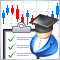如何创建订购交易机器人的需求规范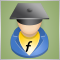在 EA 交易代码中实现指标的计算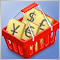货币对形态测试: 实际应用和真实交易视角。 第四部分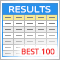可视化使用选定标准优化的结果